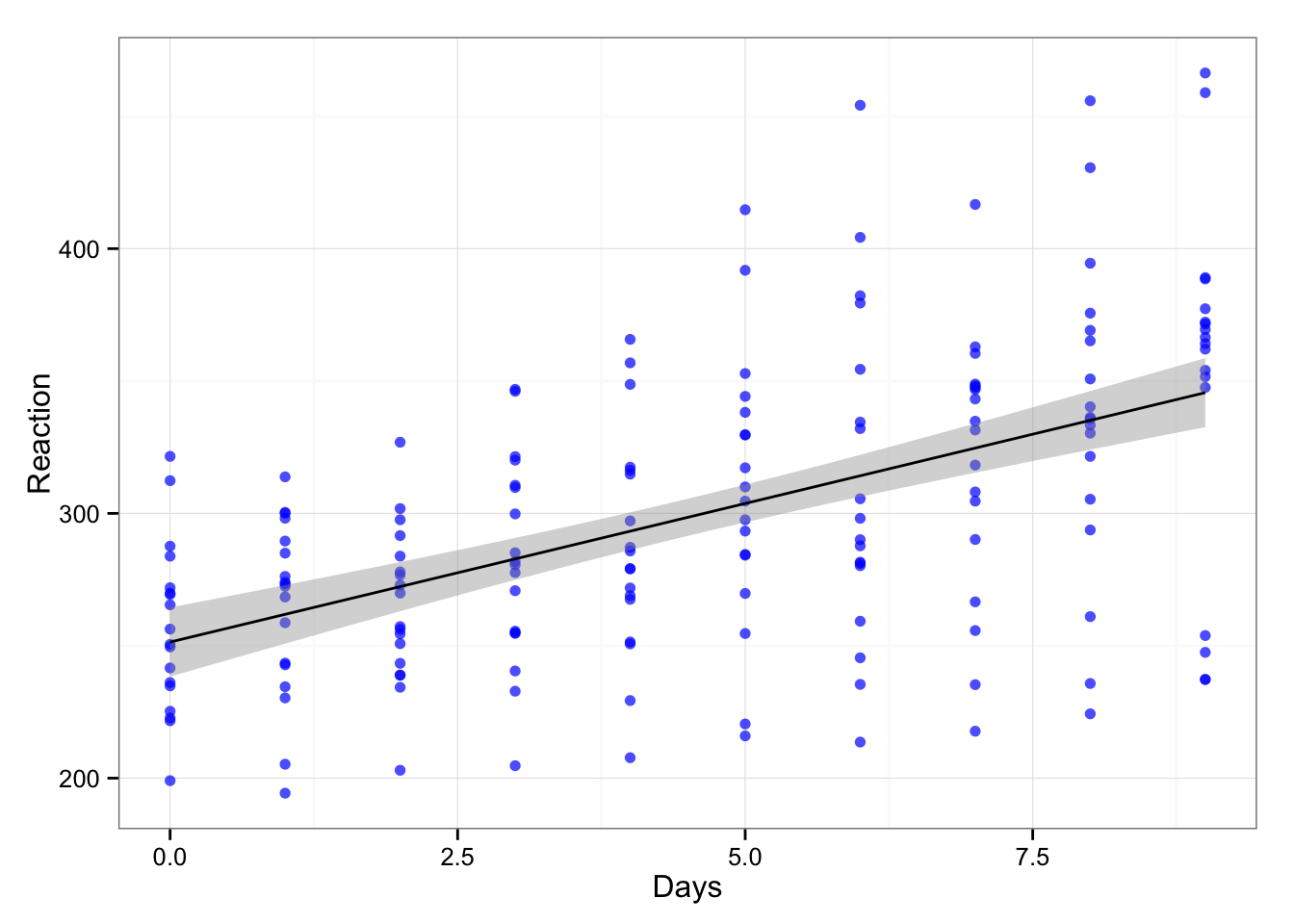I just started reading more about mixed-models (multilevel/hierarchical) and will use this as a playbook. Mostly becaus I learn the best by experimenting with the data and I suggest everyone to try to do the same. So please note that this is just a published playbook - if you find it useful, great, if you find some errors, please let me know, if you find it useless, well, disregard it.

Anyway, here is our sleep study data from lme4 package:

The data represents the average reaction time per day for subjects in a sleep deprivation study. On day 0 the subjects had their normal amount of sleep. Starting that night they were restricted to 3 hours of sleep per night. The observations represent the average reaction time on a series of tests given each day to each subject.

Basically repeated measures design. Let’s see the relationship between days and reaction time.

``````gg <- ggplot(sleepstudy, aes(x = Days, y = Reaction))
gg <- gg + geom_point(color = "blue", alpha = 0.7)
gg <- gg + geom_smooth(method = "lm", color = "black")
gg <- gg + theme_bw()
gg``````If we create simple linear model we get the following

``````model1 <- lm(Reaction ~ Days, sleepstudy)
summary(model1)``````
``````##
## Call:
## lm(formula = Reaction ~ Days, data = sleepstudy)
##
## Residuals:
##      Min       1Q   Median       3Q      Max
## -110.848  -27.483    1.546   26.142  139.953
##
## Coefficients:
##             Estimate Std. Error t value Pr(>|t|)
## (Intercept)  251.405      6.610  38.033  < 2e-16 ***
## Days          10.467      1.238   8.454 9.89e-15 ***
## ---
## Signif. codes:  0 '***' 0.001 '**' 0.01 '*' 0.05 '.' 0.1 ' ' 1
##
## Residual standard error: 47.71 on 178 degrees of freedom
## Multiple R-squared:  0.2865, Adjusted R-squared:  0.2825
## F-statistic: 71.46 on 1 and 178 DF,  p-value: 9.894e-15``````

And our coefficients are the following:

``coef(model1)``
``````## (Intercept)        Days
##   251.40510    10.46729``````

What we learned from the model is that days with sleep deprivations are negativelly affecting reaction time. The 95% CIs are:

``round(confint(model1), 2)``
``````##              2.5 % 97.5 %
## (Intercept) 238.36 264.45
## Days          8.02  12.91``````

But we included all the subjects into one bin and there is probably variation within the subjects. Let’s plot the trellis graph across the subjects:

``````gg <- gg + facet_wrap(~Subject)
gg``````